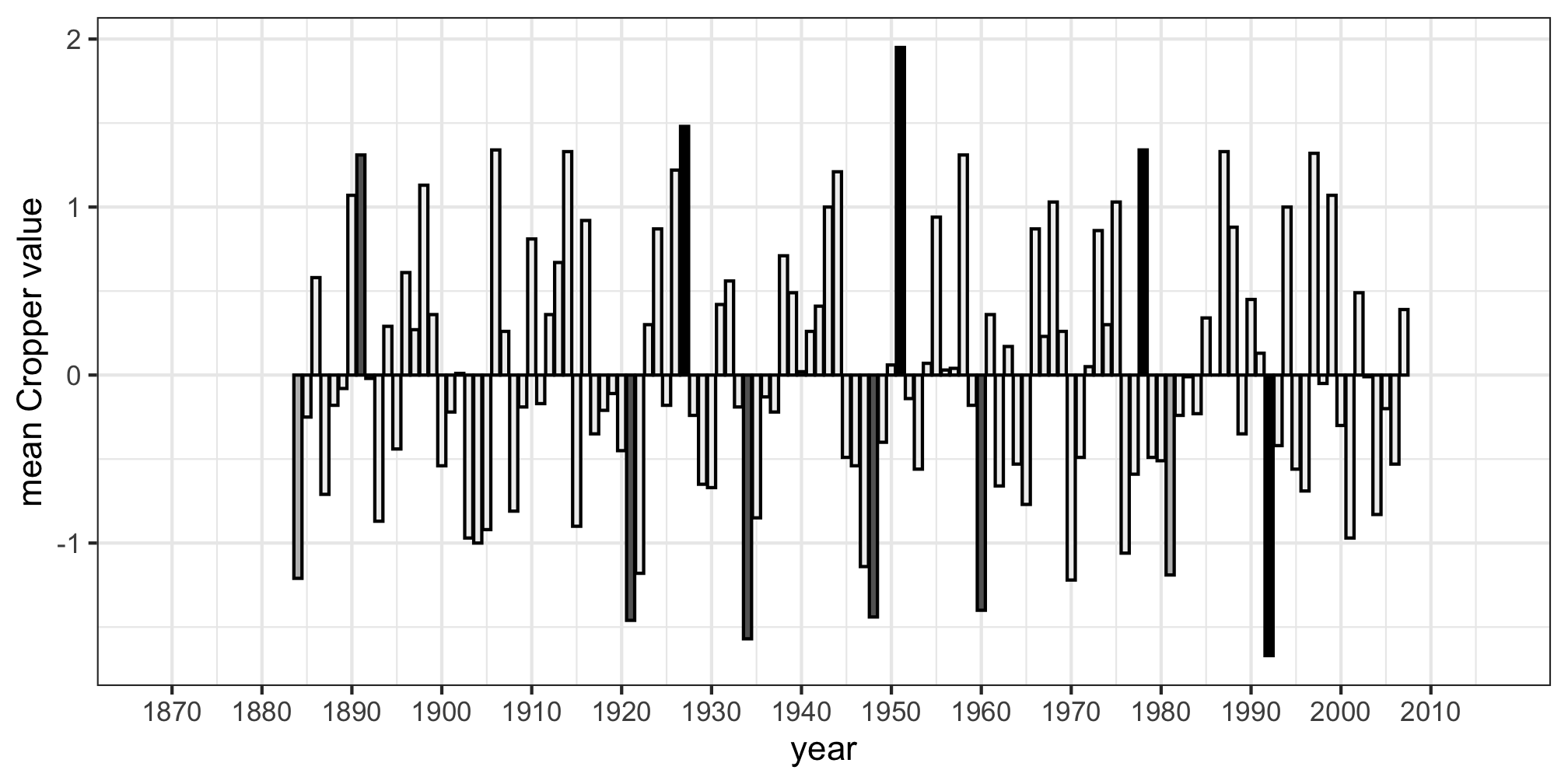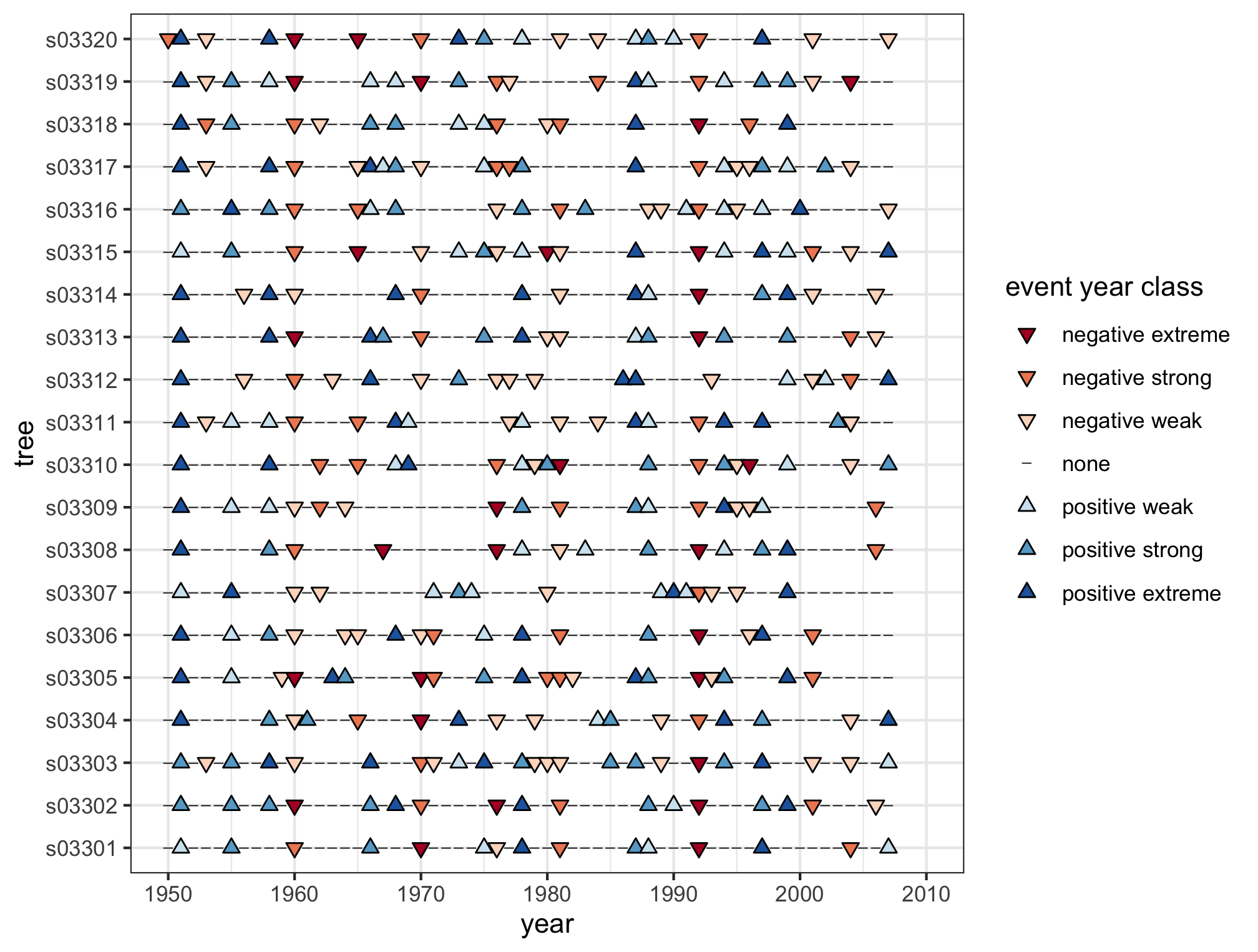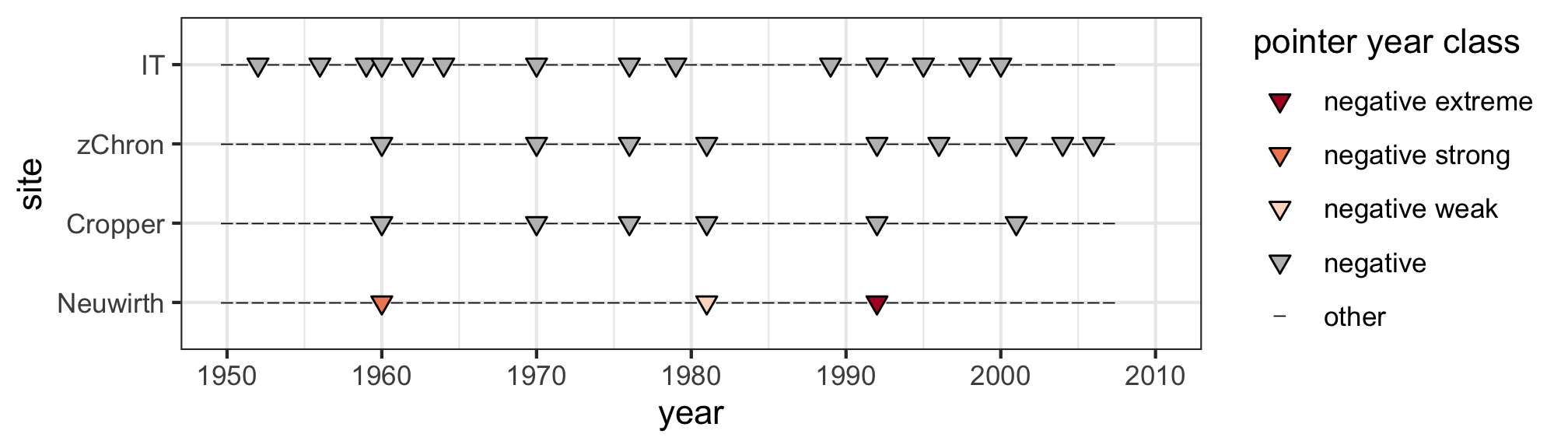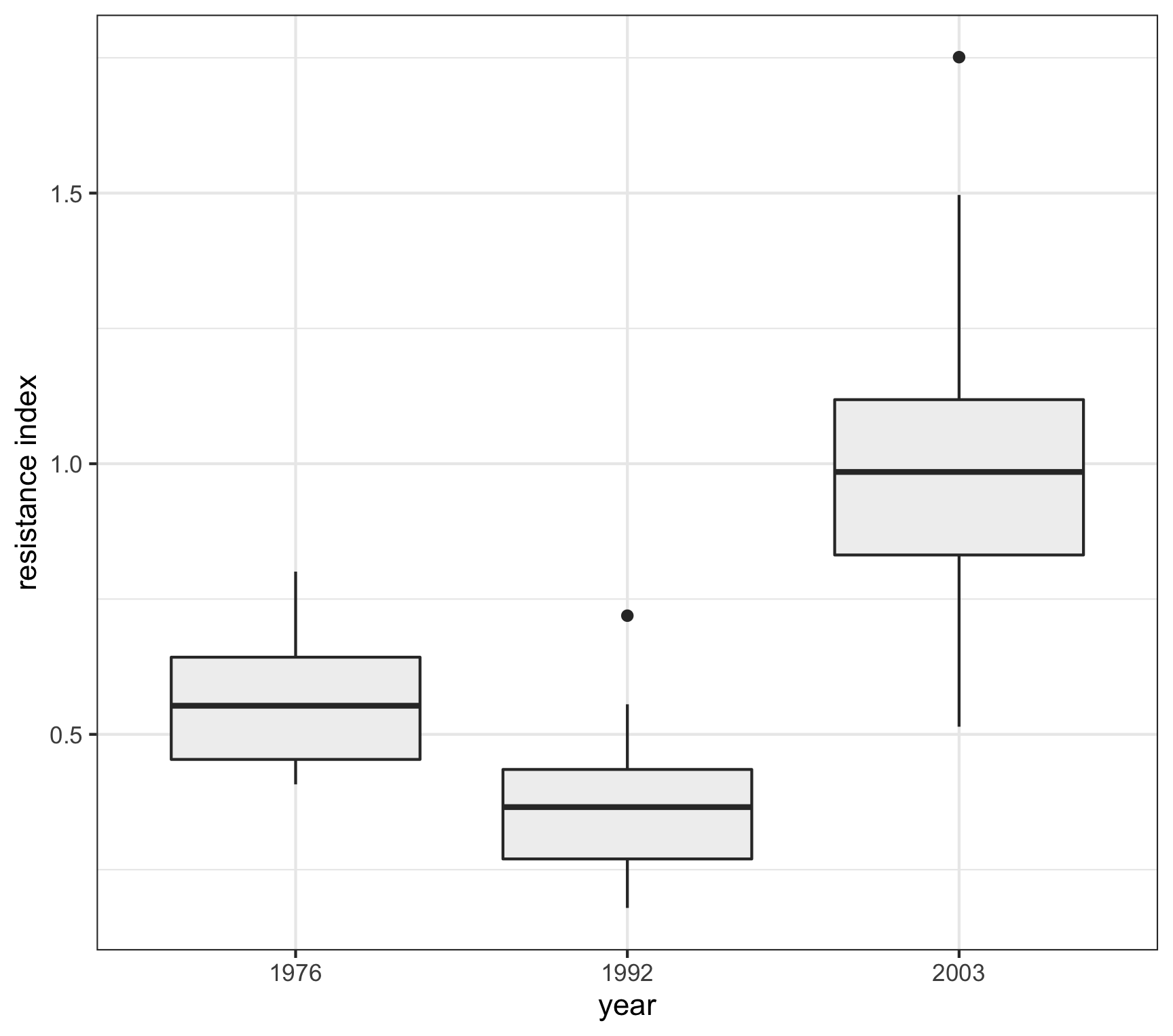# Introduction to pointRes

The package pointRes helps to analyze event years, pointer years and different resilience indices for tree-ring datasets (e.g., tree-ring width or basal area increment) by offering highly flexible calculating and plotting functions. The analysis of pointer years and resilience indices provides quantitative information on growth responses of trees to extreme events as well as on their ability to retain growth levels prior to disturbance. In the face of climate change, with a projected increase in intensity and frequency of extreme events, such information is highly important.

The package contains functions to calculate and plot event and pointer years. Event years are years with a remarkable growth increase or decrease at the individual-tree level, whereas the term pointer year refers to years with remarkable growth responses at the stand level (Schweingruber et al. 1990). To identify event and pointer years, different methods have been developed. Most established are methods of the type (i) normalization in a moving window, and (ii) relative growth change. pointRes contains customizable functions for both methods, as well as for the methods (iii) z-transformation of a site chronology and (iv) interval trend. All functions are illustrated in this vignette.

Further, pointRes contains functions to calculate and plot measures of tree resilience after Lloret et al. (2011; i.e. resistance, recovery, (relative) resilience), Thurm et al. (2016; recovery period, total growth reduction) and Schwarz et al. (2020; average growth reduction, average recovery rate). Resistance is the ratio between the growth during and before an extreme event, recovery the ratio between the growth after and during an extreme event, resilience the ratio between the growth after and before an extreme event, and relative resilience the resilience weighted by the growth decrease experienced during an extreme event. Recovery period (or: ‘growth recovery time’) is the time needed to reach pre-disturbance growth levels again. Total growth reduction reflects the cumulative growth reduction in the year of disturbance as well as the associated years in the recovery period. Average growth reduction is the total growth reduction divided by the length of the recovery period. Average recovery rate is the mean percentual recovery over the recovery period.

Detailed information on pointRes can be found on the help pages of the functions, as well as in Van der Maaten-Theunissen et al. (2015). Jetschke et al. (2019) may be consulted for more detailed information on the characteristics and use of pointer-year detection methods.

Throughout this vignette we will use the data set s033 for illustration purposes. The data set comes with the package and presents tree-ring series for 20 European beech (Fagus sylvatica L.) trees from the forest reserve Schneetal, Bavaria, Germany (Príncipe et al. 2017).

library(pointRes)
data(s033) # the result of s033 <- read.rwl('s033.rwl') - a function of the dplR package

## Event and pointer years

### Calculating event and pointer years

#### Normalization in a moving window

The normalization in a moving window method was initially proposed by Cropper (1979) for skeleton plotting. It produces time series of so-called Cropper values (C) by normalizing individual tree-ring indices in a moving window of specified width with each year placed as central point. Thresholds on these Cropper values, which basically reflect the number of standard deviations (SD) from the local mean, are used to identify event years (e.g., |C| > 0.75 SD). In a next step, a threshold on the percentage of trees showing a negative or positive event year can be used to define negative or positive pointer years. A variant of this method, proposed by Neuwirth et al. (2007), distinguishes three intensity classes for event and pointer years, i.e., weak, strong and extreme. In pointRes, the function pointer.norm facilitates the normalization in a moving window method, sensu Cropper and sensu Neuwirth. Input data should be detrended tree-ring series.

detr_s033 <- detrend(s033, method = "Spline", nyrs = 30)
pyc <- pointer.norm(detr_s033, period = NULL, window = 13, method.thresh = "Cropper", C.thresh = 0.75, series.thresh = 75, make.plot = FALSE)
pyn <- pointer.norm(detr_s033, period = NULL, window = 13, method.thresh = "Neuwirth", N.thresh = c(1, 1.28, 1.645), series.thresh = 75, make.plot = FALSE)

#### Relative growth change

The relative growth change method, also referred to as abrupt growth change method, relates tree growth in a particular year to the average growth of a specified number of preceding years (Schweingruber et al. 1986; 1990). Similar to the normalization in a moving window method, thresholds on relative growth changes can be set to define event years, and on a minimum percentage of trees showing an event year to define pointer years. In pointRes, the function pointer.rgc facilitates the relative growth change method.

rgc <- pointer.rgc(s033, period = NULL, nb.yrs = 4, rgc.thresh.pos = 60, rgc.thresh.neg = 40, series.thresh = 75, make.plot = FALSE)

#### Z-transformation of a site chronology

This method calculates pointer years by developing and z-transforming a site chronology. Pointer years are defined over a threshold on the minimum of standard deviations that the site chronology should deviate in a particular year. To test whether the z-transformed chronology significantly differs from the selected threshold value, t-tests can be performed (robust or non-robust, depending whether or not a biweight robust mean was used in chronology building). In pointRes, the function pointer.zchron facilitates the z-transformation of a site chronology method.

detr_s033 <- detrend(s033, method = "Spline", nyrs = 30)
pz <- pointer.zchron(detr_s033, period = NULL, bi.weight = TRUE, z.thresh = 1, t.Test = FALSE, make.plot = FALSE) 

#### Calculating the percentage of rising (and descending) intervals

The function calculates year-to-year growth changes for individual tree-ring series and defines interval trends for (the population of) trees using the pointer interval method according to Schweingruber et al. (1990), which is also extensively described in Jetschke et al. (2019). For each tree and year, the interval trend is defined as 1 if a relative change exceeds a certain positive threshold, as 0 if a relative (negative) change falls below minus that threshold and 0.5 otherwise; defaults to 0%. The interval trend for a population is defined as the average interval trend of the individual trees. A year is considered a negative (or positive) pointer year if the percentage of trees showing a decreasing (or increasing) trend exceeds a threshold (defaults to 95%).The minimum percentual growth change and the minimum percentage of trees that should display a negative (or positive) trend for that year to be considered as pointer year, can be adjusted.

it <- interval.trend(s033, period = NULL, trend.thresh = 0, IT.thresh = 95, make.plot = FALSE) 

### Plotting event and pointer years

If the argument make.plot in the functions pointer.norm, pointer.rgc, pointer.zchron and interval.trend is set TRUE, a plot is created. Depending on the function, a bar plot displaying mean annual Cropper values (pointer.norm) or mean annual growth deviations (in percentage; pointer.rgc), or a line plot displaying z-scores (pointer.zchron) or mean interval-trend values (interval.trend), is created. Pointer years are indicated with different symbols and (or) colors For the pointer.norm method Neuwirth, weak, strong and extreme pointer years are defined based on the most common event year class.

detr_s033 <- detrend(s033, method = "Spline", nyrs = 30)
pyn <- pointer.norm(detr_s033, method = "Neuwirth", make.plot = TRUE)Besides bar and line plots, dot plots for event years (i.e. for single trees) and pointer years (for multiple sites) can be created using the functions event.plot and pointer.plot, respectively, whereby event-year plots can only be created with output of the functions pointer.norm and pointer.rgc, given the conceptually different approaches of pointer.zchron and interval.trend. An example of a dot plot with event years, as defined using pointer.norm method Neuwirth, is provided below.

event.plot(pyn, period = c(1950, 2007), x.tick.major = 10, x.tick.minor = 5)The optional argument sign allows to display only positive, negative or both positive and negative event/pointer years. A dot plot comparing negative pointer years detected by different methods can, for example, be created as follows:

pointer.plot(list(pyn,pyc,pz,it), sign = "neg", period = c(1950, 2007), labels = c("Neuwirth","Cropper","zChron","IT"))
## Warning in pointer.plot(list(pyn, pyc, pz, it), sign = "neg", period = c(1950, :
## 'list.sites' contains output of multiple functions to calculate pointer years.
## When performing a method comparison, please ignore this warningThe resulting warning is just a slight reminder that different methods of pointer year calculation have been applied to the datasets considered in pointer.plot.

## Indices of tree resilience

The function res.comp calculates the resilience indices after Lloret et al. (2011), Thurm et al. (2016) and Schwarz et al. (2020). The argument nb.yrs specifies the number of years for pre- and post-disturbance periods to be considered in calculating resilience components after Lloret et al. (2011). With max.yrs.rec the maximum length of the recovery period to be considered can be defined.

res <- res.comp(s033, nb.yrs = c(4,4), max.yrs.rec = 10)

The output of res.comp is a list of matrices for the various resilience indices.

The function res.plot uses the output of res.comp to create a box plot for selected resilience indices. These box plots show the full range of variation for user-defined years. Box plots are only displayed for years for which indices are available for at least five tree-ring series, as this is the number of statistics that a box plot represents in its simplest form.

res.plot(res, select.yr = c(1976, 1992, 2003), param = "resist")
## Please note that the number of series upon which individual boxplots are based can be queried by calling the component 'nb.series' from the res.comp output. Numbers may deviate between years and indices, depending on tree-specific growth behaviour as well as characteristics inherent to the calculation methods## Citing pointRes and R

When using the pointRes package in your work, we ask you to cite pointRes and R appropriately. Execute the function citation() for information on how to cite the package and R.

citation()
##
## To cite R in publications use:
##
##   R Core Team (2022). R: A language and environment for statistical
##   computing. R Foundation for Statistical Computing, Vienna, Austria.
##   URL https://www.R-project.org/.
##
## A BibTeX entry for LaTeX users is
##
##   @Manual{,
##     title = {R: A Language and Environment for Statistical Computing},
##     author = {{R Core Team}},
##     organization = {R Foundation for Statistical Computing},
##     year = {2022},
##     url = {https://www.R-project.org/},
##   }
##
## We have invested a lot of time and effort in creating R, please cite it
## citing R packages.
citation("pointRes")
##
## To cite pointRes in publications, please use:
##
##   van der Maaten-Theunissen M, van der Maaten E, Bouriaud O (2015).
##   "pointRes: An R package to analyze pointer years and components of
##   resilience." _Dendrochronologia_, *35*, 34-38.
##   doi:10.1016/j.dendro.2015.05.006
##   <https://doi.org/10.1016/j.dendro.2015.05.006>.
##
## and:
##
##   van der Maaten-Theunissen M, Trouillier M, Schwarz J, Skiadaresis G,
##   Thurm E, van der Maaten E (2021). "pointRes 2.0: New functions to
##   describe tree resilience." _Dendrochronologia_, *70*, 125899.
##   doi:10.1016/j.dendro.2021.125899
##   <https://doi.org/10.1016/j.dendro.2021.125899>.
##
## If you use the function pointer.zchron or interval.trend, please cite
## the following publication as well:
##
##   Jetschke G, van der Maaten E, van der Maaten-Theunissen M (2019).
##   "Towards the extremes: A critical analysis of pointer year detection
##   methods." _Dendrochronologia_, *53*, 55-62.
##   doi:10.1016/j.dendro.2018.11.004
##   <https://doi.org/10.1016/j.dendro.2018.11.004>.
##
## Pointer year detection is a delicate issue. Please consult the
## following publication:
##
##   Jetschke G, van der Maaten E, van der Maaten-Theunissen M (2023).
##   "Pointer years revisited: Does one method fit all? A clarifying
##   discussion." _Dendrochronologia_, *78*, 126064.
##   doi:10.1016/j.dendro.2023.126064
##   <https://doi.org/10.1016/j.dendro.2023.126064>.
##
## To see these entries in BibTeX format, use 'print(<citation>,
## bibtex=TRUE)', 'toBibtex(.)', or set
## 'options(citation.bibtex.max=999)'.